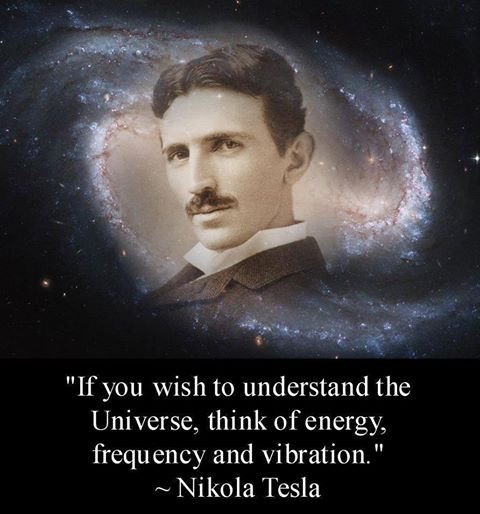369(source unknown) (click to enlarge)

"If you only knew the magnificence of the 3, 6 and 9, then you would have a key to the universe." Nikola Tesla (source of this quote unknown which puts in question whether Tesla even said this).

I have found no other reference to this quote attributed to Nikola Tesla. Are there any? If you know the source of this quote and/or have more Tesla materials on this idea please contact me.

However, what I have found are numerous references within the Keely materials. Russell also mentions the importance of threes. Dale Pond

The 3, 6 and 9 idea has been partially explained in Laws of Being - Annotated and more completely explained with considerable detail in Dale Pond's new book Keely's Laws of Being - The Nature and Dynamics of Vibration

"The relative frequency of all sympathetic streams is in the ratio 3:6:9. Those whose relative frequencies are 3:9 are mutually attractive, while those having the relation of 6:9 are mutually repellant." [Keely, SYMPATHETIC STREAMS - Snell, LAWS OF MOLECULAR BEING, Sympathetic Stream, Modes of Vibration, Modes of Vibration - Annotated]

369 = Powers of 2 and Volumes One of the basic fundamentals of vibration is the formation of its octave or doubling of frequency and simultaneous halving of its wave length. These features are functions of 2 (multiplication) and its reciprocal 1/2 (division).

One of the basic fundamentals of sound or energy is manifesting as (geometric) volume - a function of cubing (n^3).

Merging these two fundamental principles we arrive at a series of cubes derived from powers of two. The first few powers of two that create cubes are

2^3 = 8 units of volume, 2^6 = 64 units of volume and 2^9 = 512 units of volume. [see Figure 6.17 - Areas and Volumes - Relations and Proportions, Figure 6.19 - Sphere to Cube - Relations and Proportions, Figure 12.09 - Dimensions and Relationships, Table 12.02 - Length Area and Volume Math]

In the above we see another correlation between Russell’s philosophy and Keely’s science.

2^0=1 2^1=2 2^2=4 2^3=8 2^4=16 2^5=32 2^6=64 2^7=128 2^8=256 2^9=512Area and Volume Relations and Ratios
[Dale Pond, October, 10, 2017]
"All manifestations of Energy are modes of vibration:

1: RADIATING, ENHARMONIC, POSITIVE ATTRACTION, CELESTIAL; Attracted to the external Universe.

2: FOCALIZING, HARMONIC, NEGATIVE ATTRACTION, TERRESTRIAL; The intensification of individuality or materiality of matter.

3: DOMINANT; That controlling tendency governing the ascendancies of the first two.

All three of these (modes) must be present in every flow of energy and are always present in the ratio 3:6:9." [Snell Manuscript]

"The sympathetic acoustic impulses are: "the DOMINANT - a diatonic third - "the HARMONIC - the connective "sixth" - and "the ENHARMONIC - or diminished seventh - which Keely calls a ninth -

"inducing "infinite trajective velocity" or "neutral radiation" from neutral centers. [Snell Manuscript]

"The vitalization of the disks for the polar and depolar field is established on the ratio of thirds, sixths, and ninths; the ninths being the circuit occupied by the polar field, must represent, in the scale of vitalized focalized intensity, 100 in my system: sixths in the depolar field, or 66 2/3; and in the neutral field, or thirds, 33 1/3. The triplets must represent one true chord of equation." [The Operation of the Vibratory Circuit]

"Here we have a clue to the Thirds, Sixths and Ninths of Keely's theories, in the operations of his polar negative attractor. The conception of the Pythagoreans of music, as the principle of the creation's order, and the mainstay and supporter of the material world, is strictly in accordance with the marvelous truths which are now being unfolded to science." [14.01 - Hints from Bloomfield-Moore]

See the following entries for more detail on the enigmatic 3, 6 & 9.